# RD Sharma Solutions Class 9 Chapter 15 Areas of Parallelograms and Triangles Exercise 15.3

Here you can get free RD Sharma Solutions for Class 9 Maths Chapter 15 Areas of Parallelograms and Triangles Exercise 15.3. All RD Sharma Book Solutions are given here exercise wise for the chapter Areas of Parallelograms and Triangles. RD Sharma Solutions are helpful in the preparation of several school level, graduate and undergraduate level competitive exams. Practicing questions from RD Sharma Mathematics Solutions for Class 9 Chapter 15 Areas of Parallelograms and Triangles is proven to enhance your math skills.

 Class: Class 9th Chapter: Chapter 15 Exercise: Exercise 15.3 Name: Areas of Parallelograms and Triangles

## RD Sharma Solutions Class 9 Chapter 15 Areas of Parallelograms and Triangles Exercise 15.3

RD Sharma Class 9 Solutions Chapter 15 for Areas of Parallelograms and Triangles Exercise 15.3 are given below.

RD Sharma Solutions Class 9 Chapter 15 Area of Parallelograms and Triangles Excercise 15.3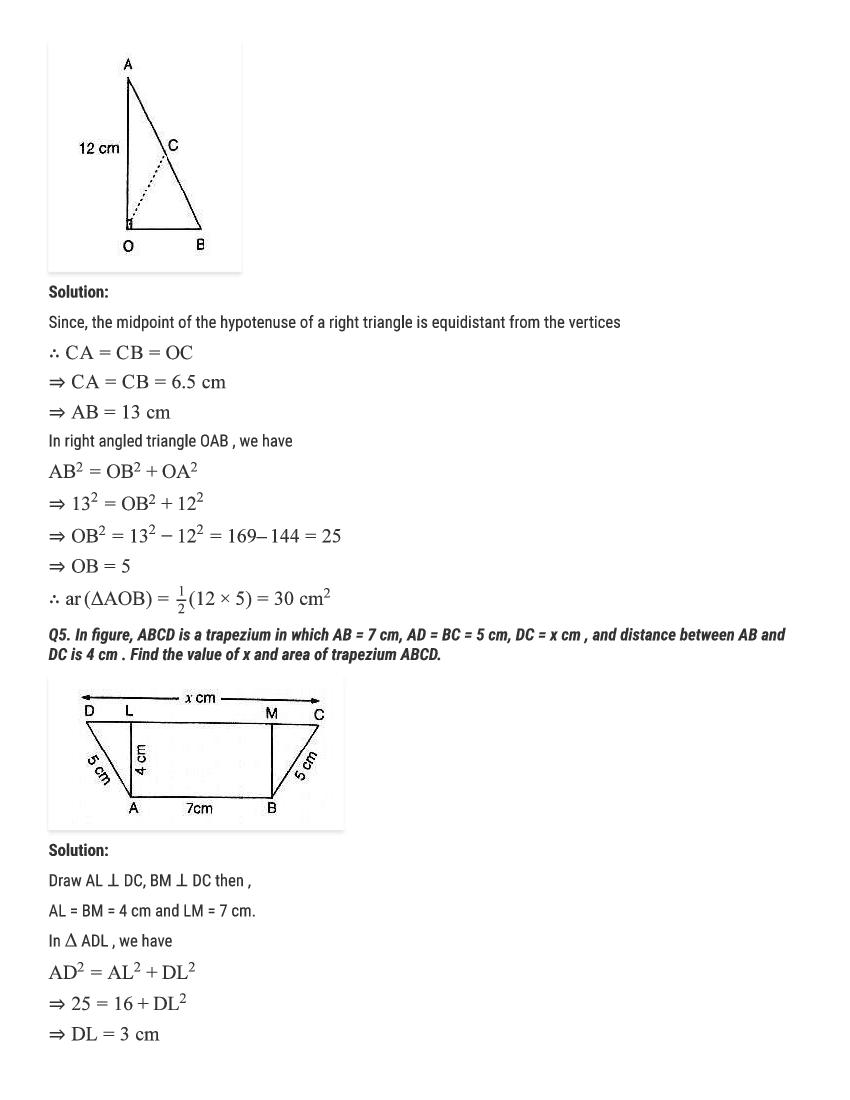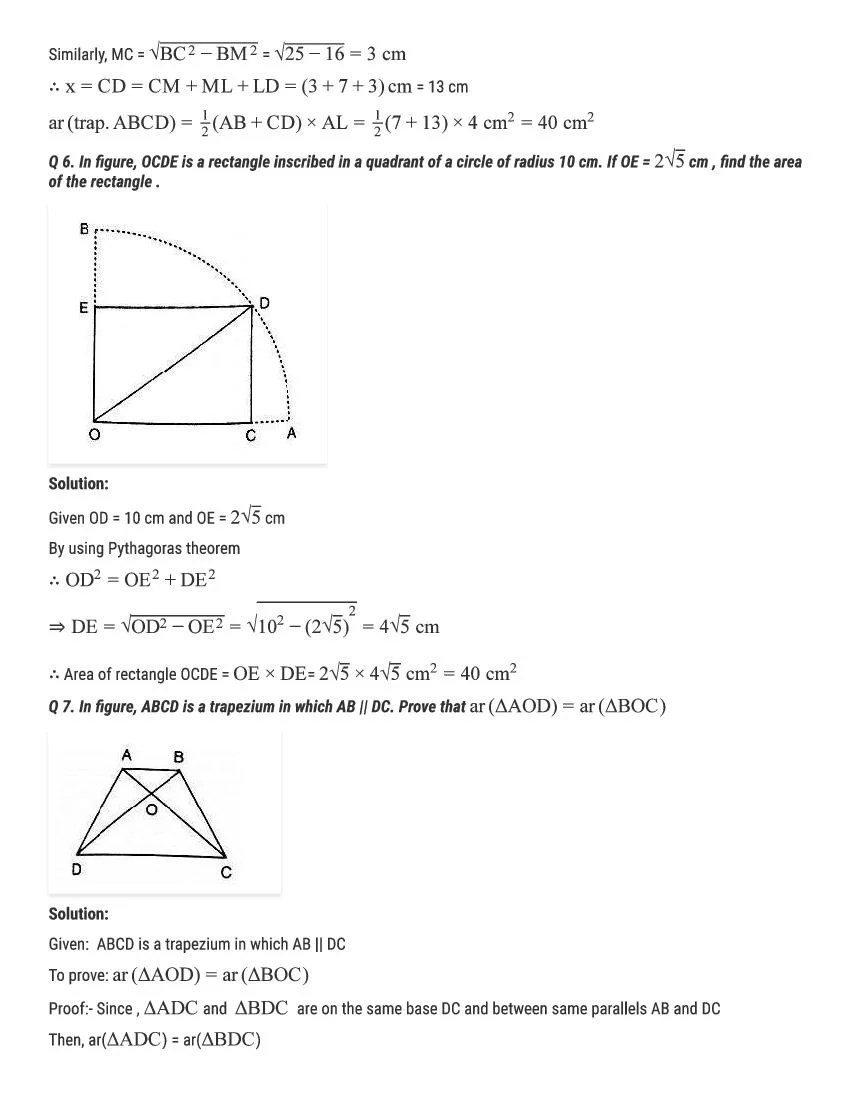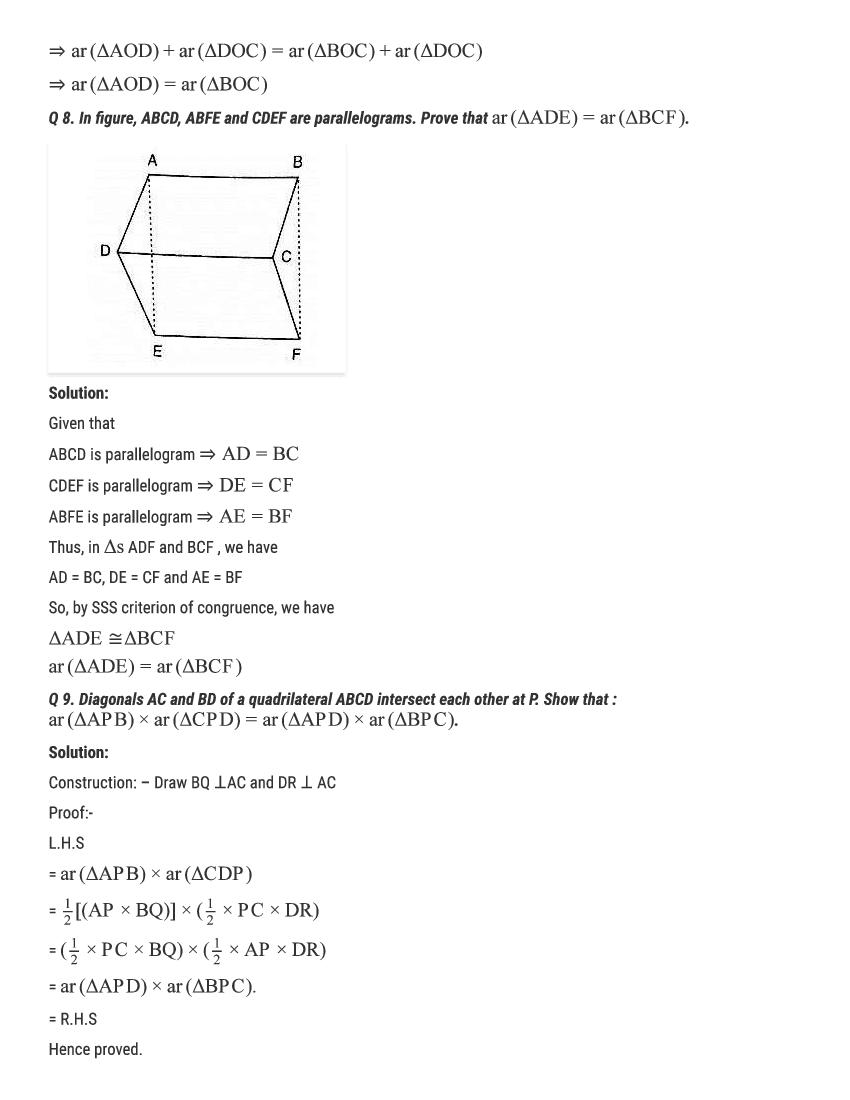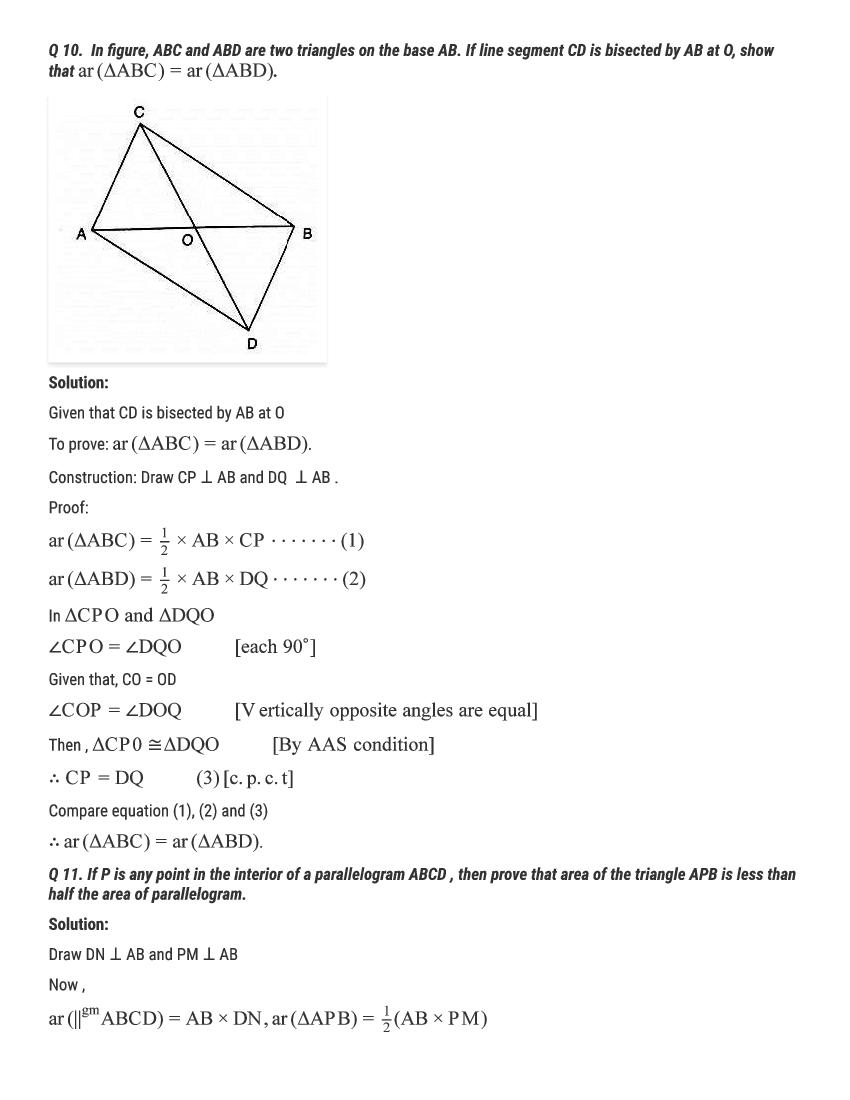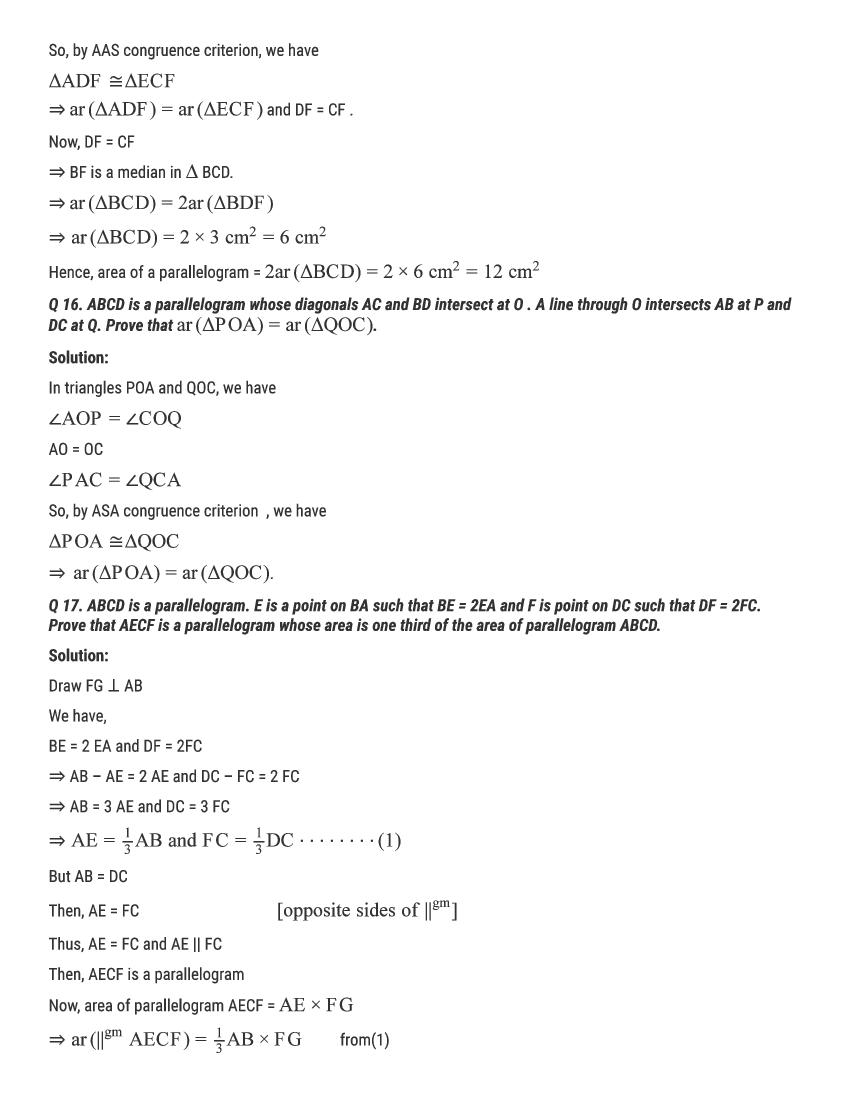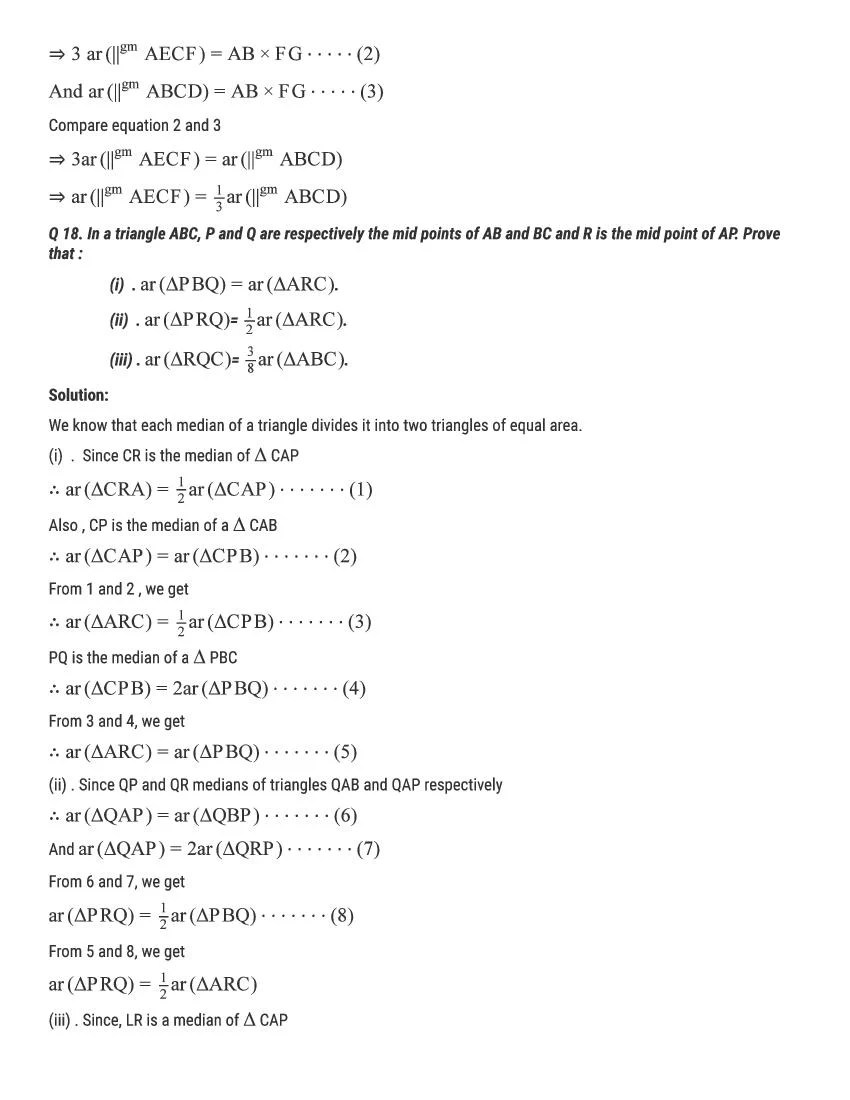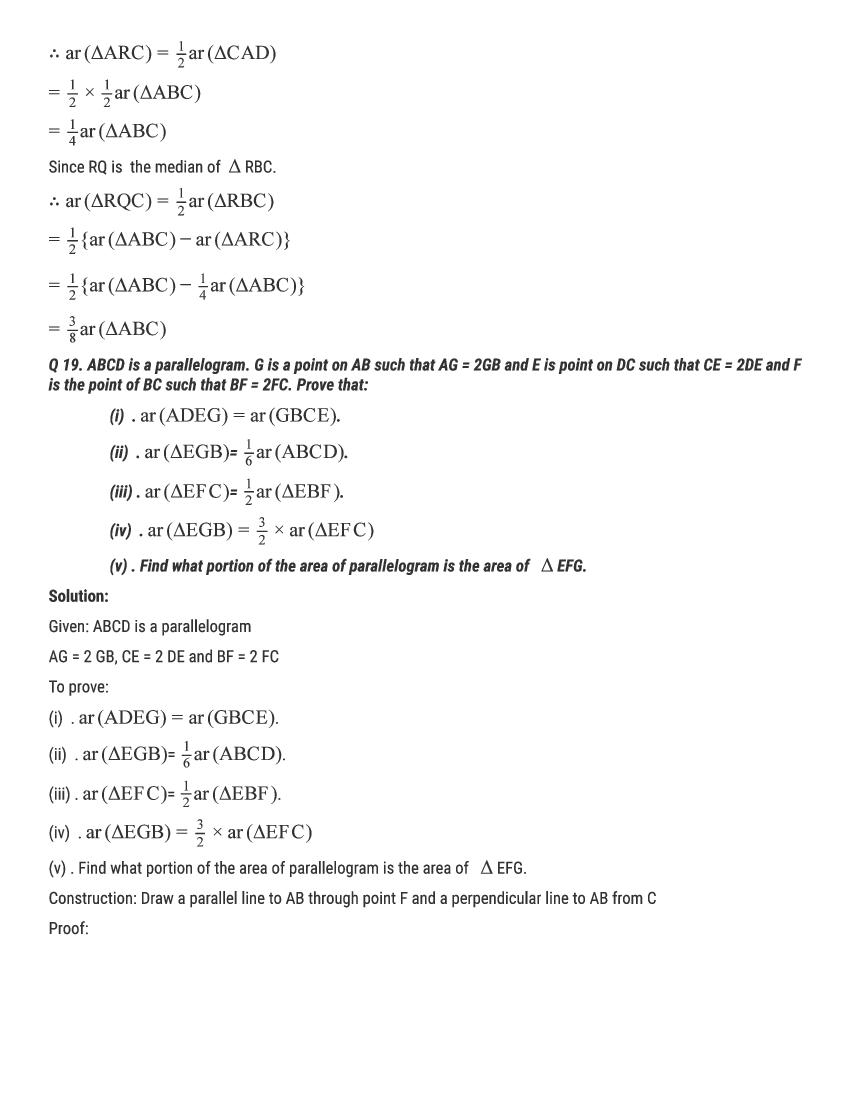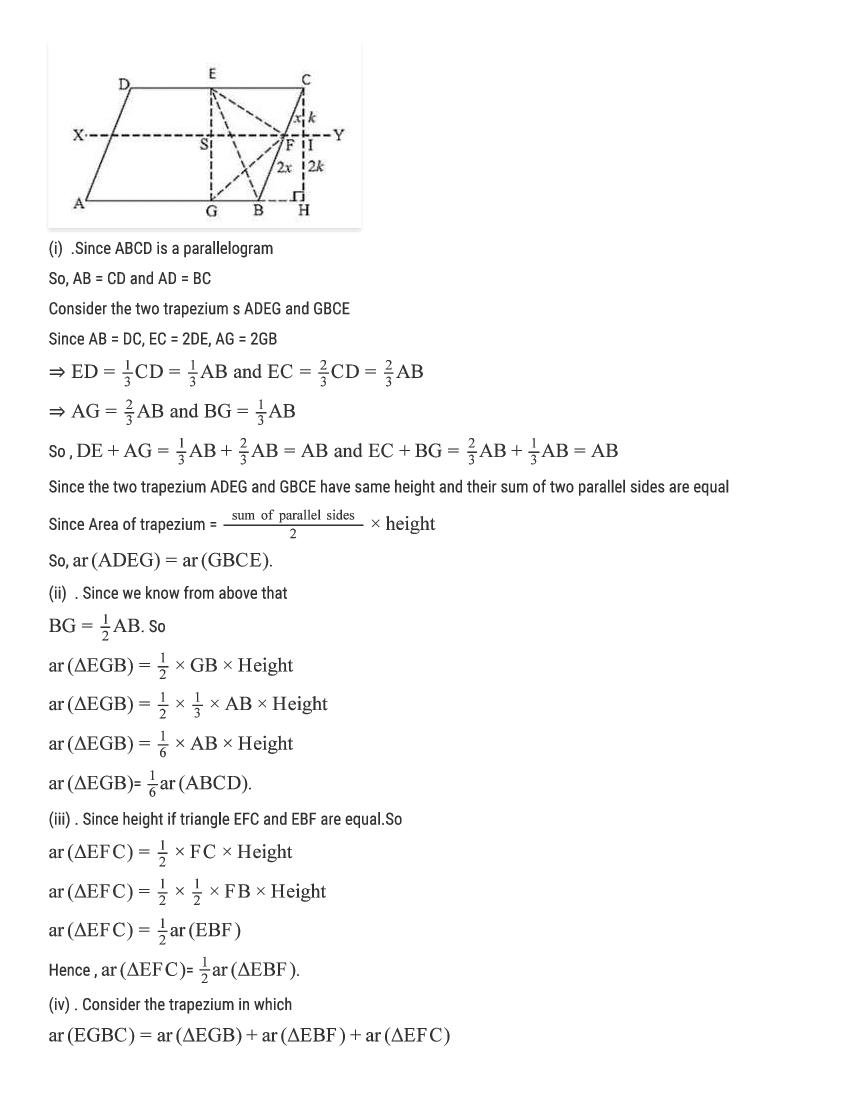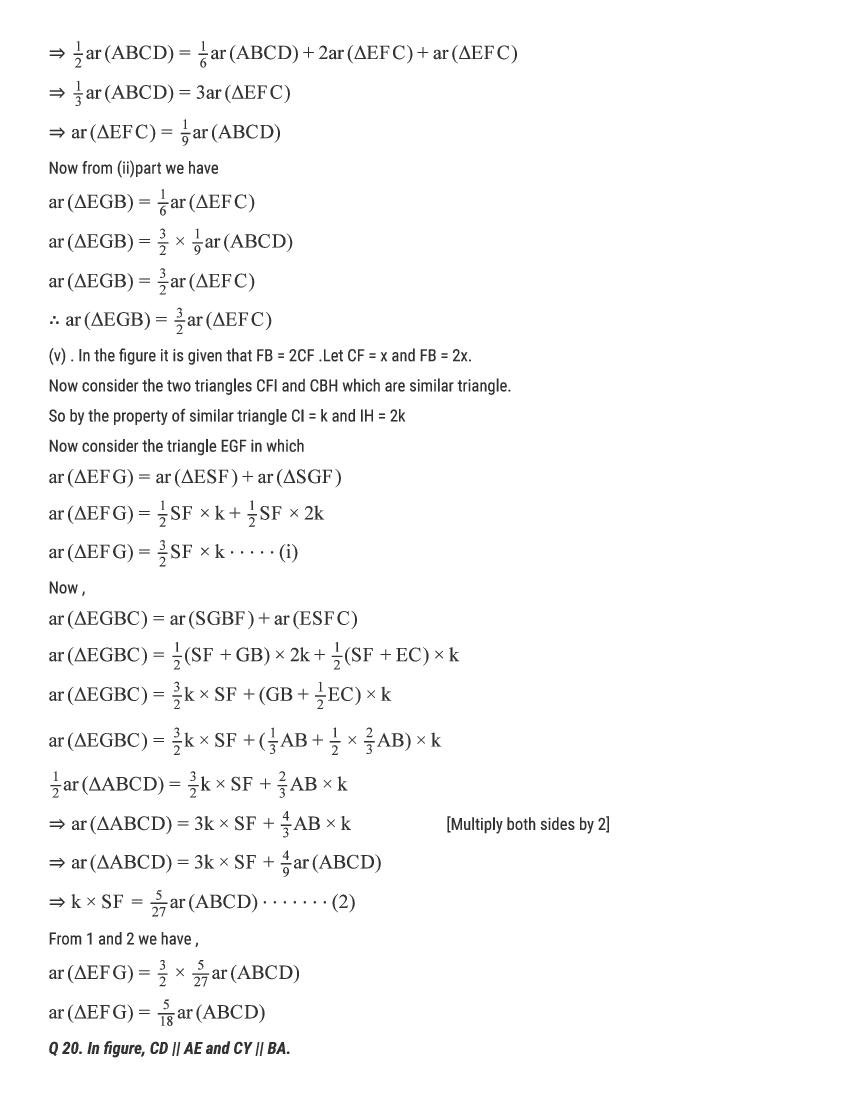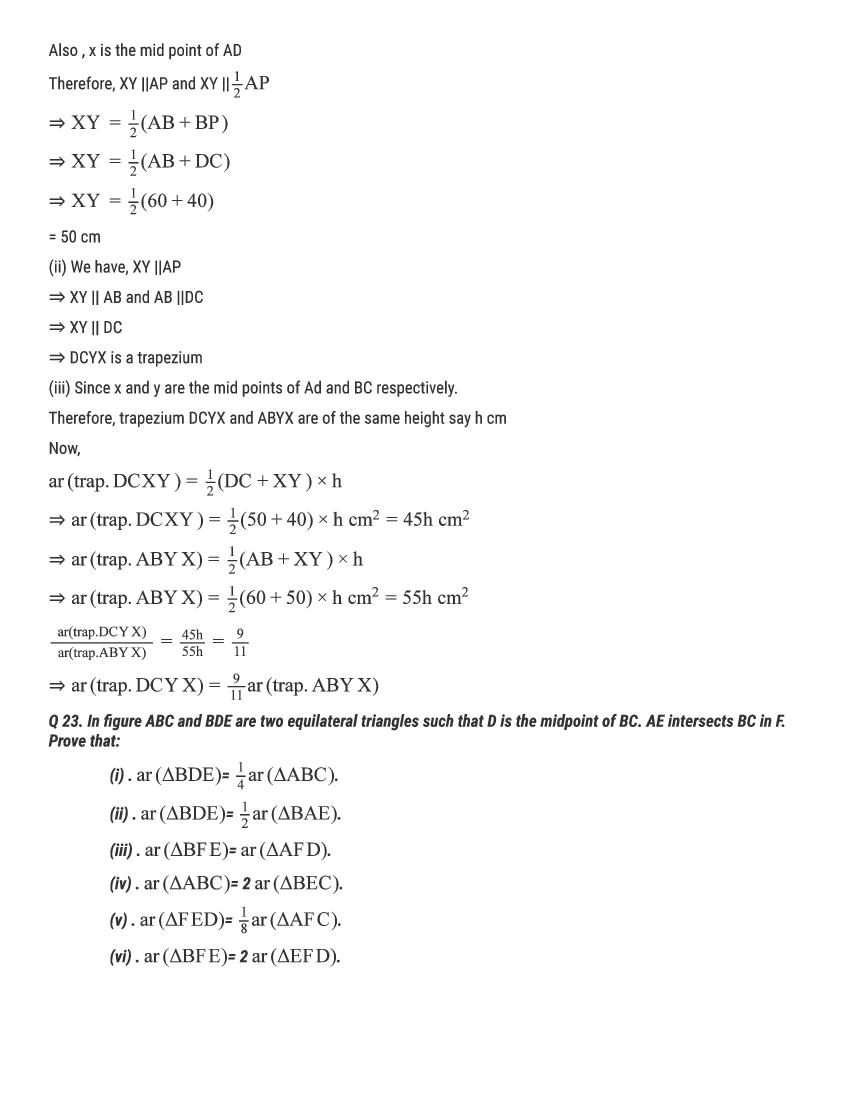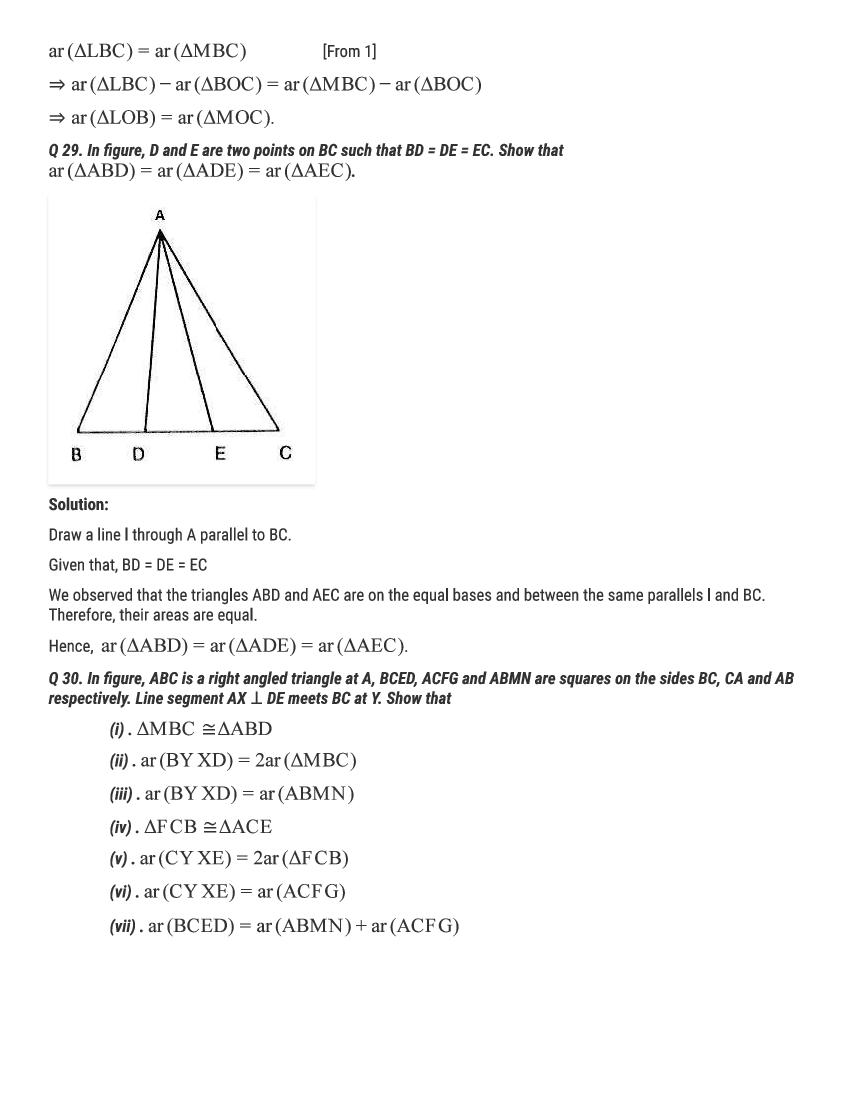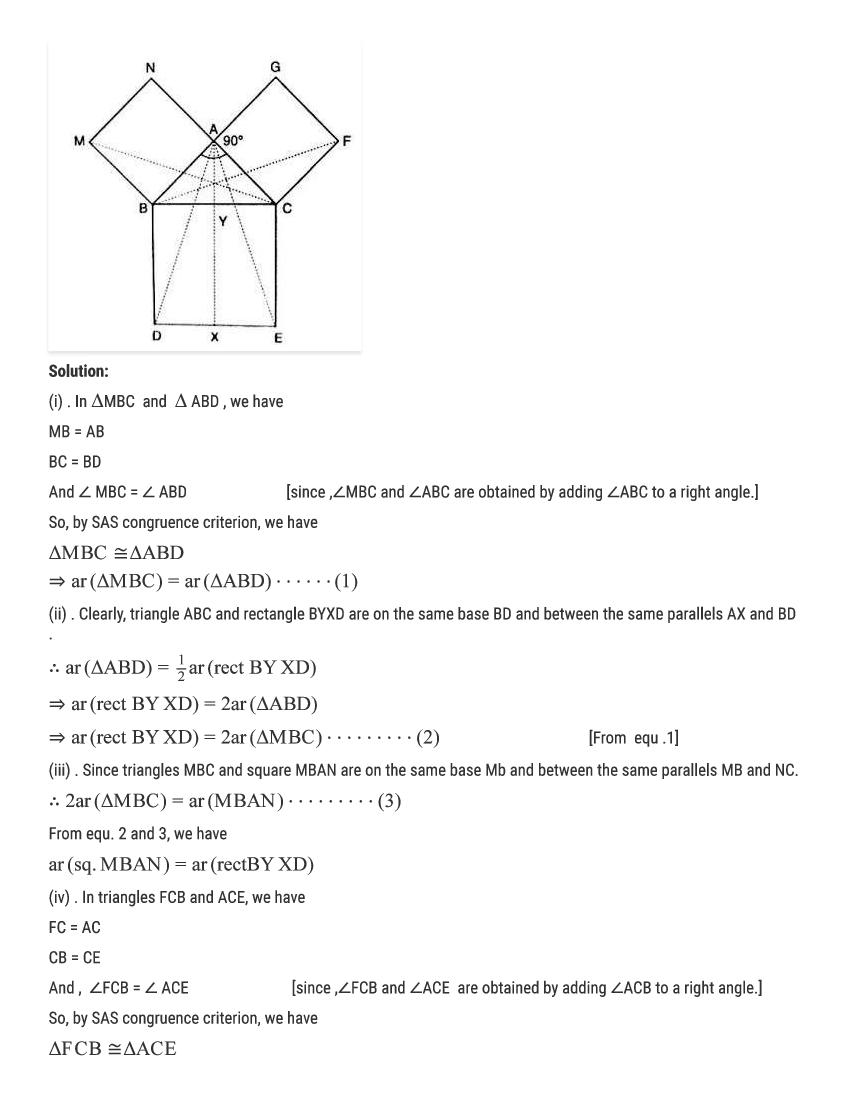RD Sharma Solutions for Class 9 Maths PDF download for Chapter 15 Areas of Parallelograms and Triangles Exercise 15.3 is available here for free.

 « Previous Next »

### RD Sharma Solutions Class 9 Chapter 15 Exercise Wise

You can also see RD Sharma Solutions of all exercises of Chapter 15 – Areas of Parallelograms and Triangles from here.

### RD Sharma Solutions Class 9 Chapter Wise

If you want RD Sharma solutions of any topic other than Chapter 15 Areas of Parallelograms and Triangles, check it from here. Mathematics by RD Sharma has all chapters that are in your Class 9 syllabus.

Click to rate this post!
[Total: 2 Average: 5]